Constructing a line segment

Practical Geometry
Serial order wise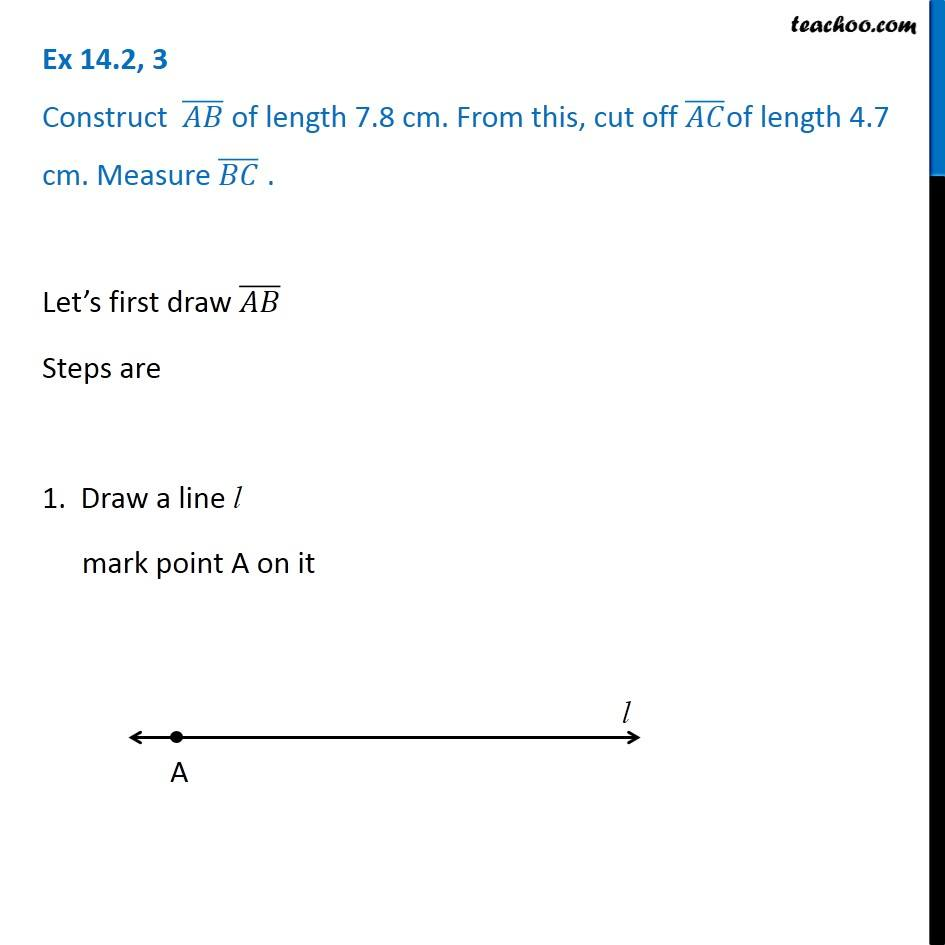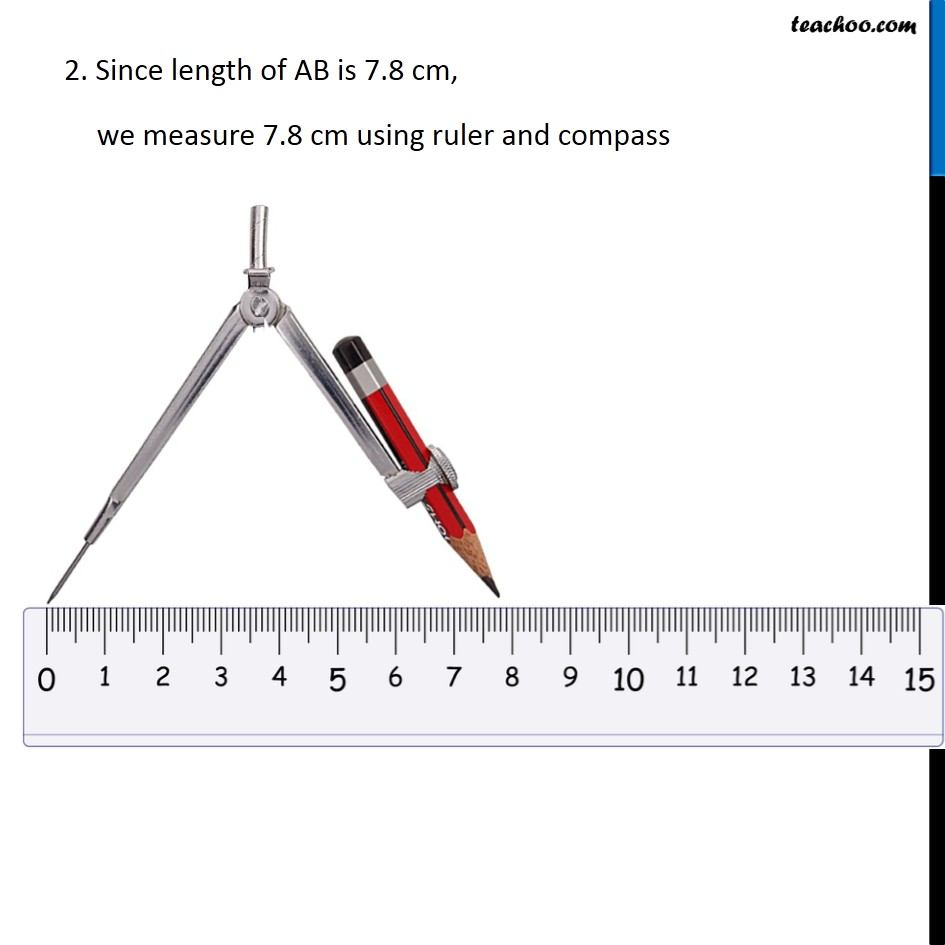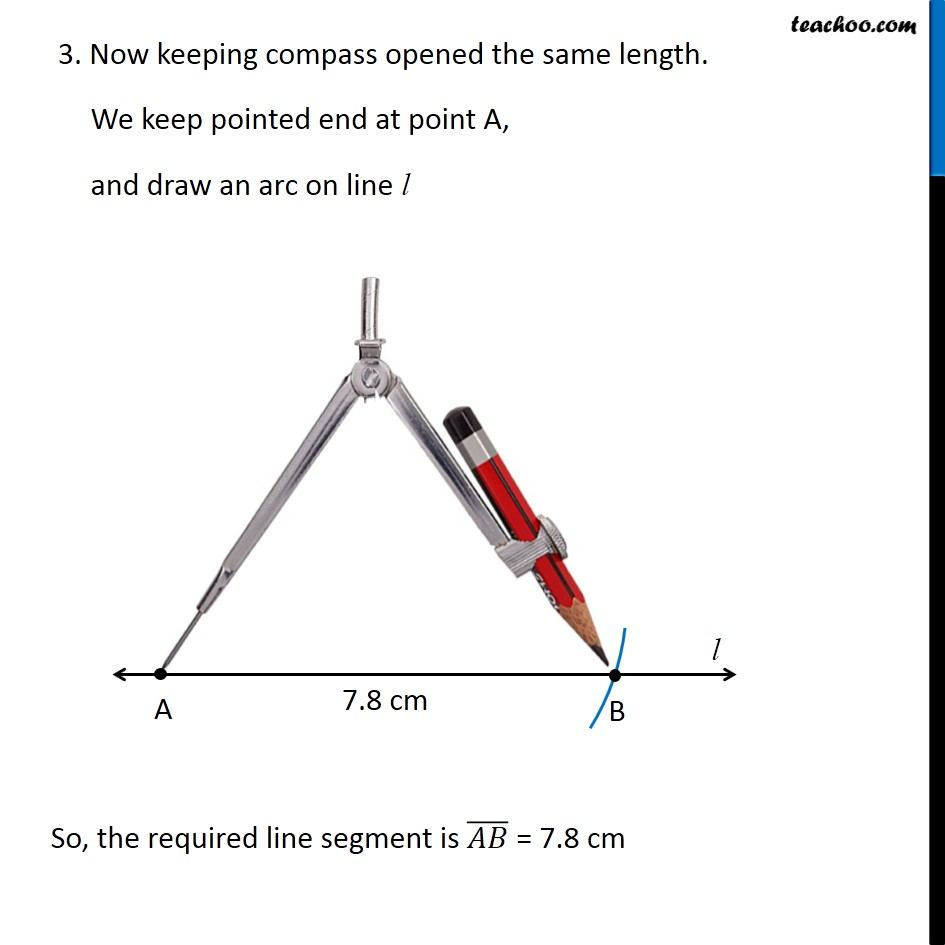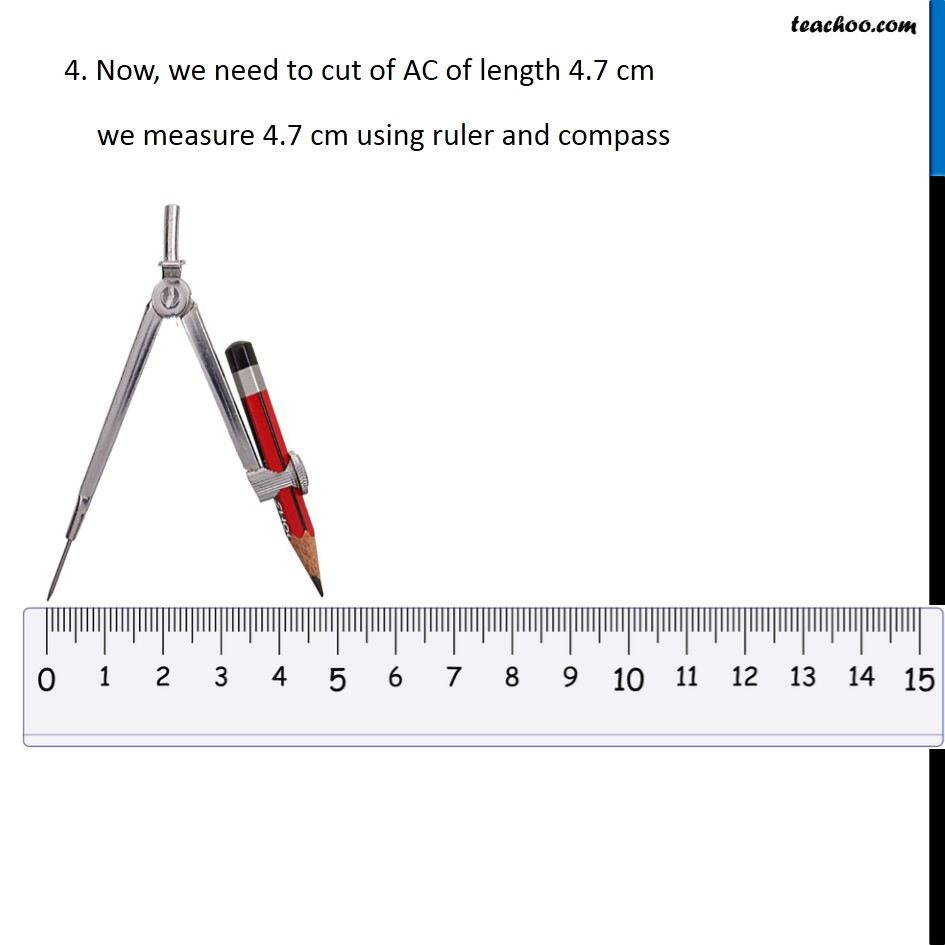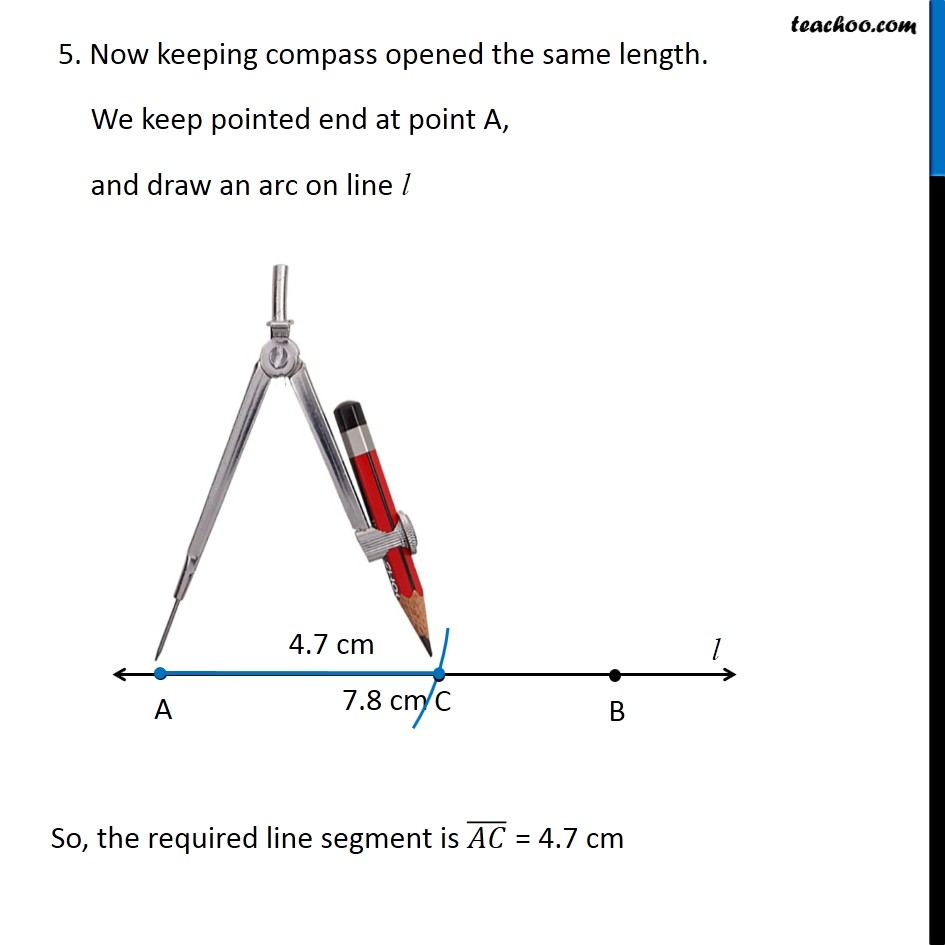Learn in your speed, with individual attention - Teachoo Maths 1-on-1 Class

### Transcript

Question 3 Construct (𝐴𝐵) ̅ of length 7.8 cm. From this, cut off (𝐴𝐶) ̅of length 4.7 cm. Measure (𝐵𝐶) ̅ . Let’s first draw (𝐴𝐵) ̅ Steps are 1. Draw a line l mark point A on it 2. Since length of AB is 7.8 cm, we measure 7.8 cm using ruler and compass 3. Now keeping compass opened the same length. We keep pointed end at point A, and draw an arc on line l So, the required line segment is (𝐴𝐵) ̅ = 7.8 cm So, the required line segment is (𝐴𝐵) ̅ = 7.8 cm 4. Now, we need to cut of AC of length 4.7 cm we measure 4.7 cm using ruler and compass 5. Now keeping compass opened the same length. We keep pointed end at point A, and draw an arc on line l So, the required line segment is (𝐴𝐶) ̅ = 4.7 cm